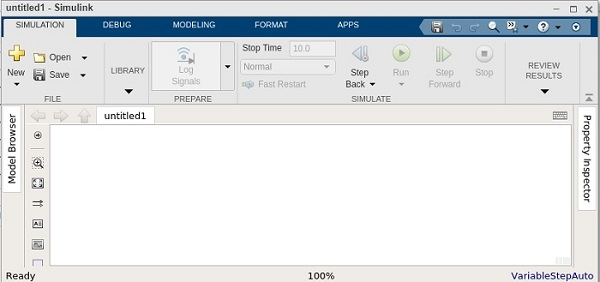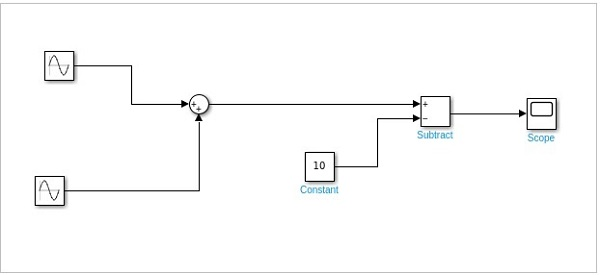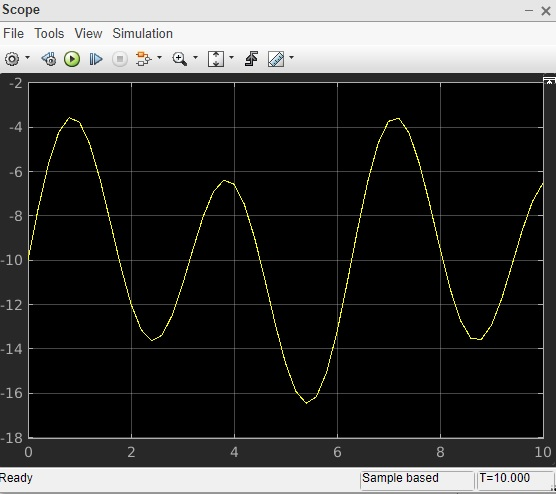# Solving Mathematical Equation

#### Data Preprocessing for Machine Learning using MATLAB

30 Lectures 4 hours

#### Image Processing Toolbox in MATLAB

17 Lectures 3 hours

#### Matlab - The Complete Course

Best Seller

37 Lectures 5 hours

In this chapter, we will solve a simple mathematical equation by using Simulink.

The equation is given below −

```y(t) = 2Sin(t) + 5Sin(2t) - 10
```

Let us create a model for the above equation. Open a blank model as shown below −Following are the steps to solve the equation −

• Get a Sine wave block. Right click and select block parameters. Select sign type as time based. Change the amplitude to 2 and frequency to 1. That will be 2Sin(t).

• Get another sine wave block. Now, set the amplitude to 5 and frequency to 2 to display 5Sin(2t). Select sign type as time based.

• Now get an add block and add both sine waves.

• Get a constant. Right click and select block parameters. Change the value from 1 to 10.

• Get a subtract block where one input will come from step 3 and another from constant i.e. step 4.

• Get a scope block and connect the input from step 6 to it.

This is how the final Simulink model looks like for the equation −

```y(t) = 2Sin(t) + 5Sin(2t) - 10
```Click on the run button to compile. Right Click on scope block to see the signal plotted.